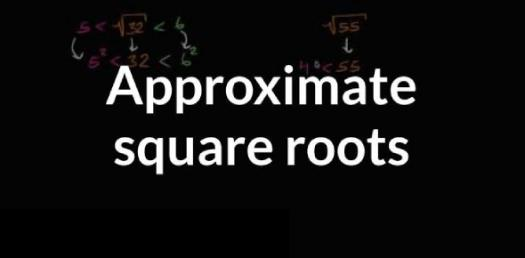# Estimating And Approximating Square Root! Math Trivia Quiz

9 Questions | Total Attempts: 230SettingsWelcome to this math trivia quiz on estimating and approximating square root! There are a lot of students who have a difficult time when it comes to solving problems such as square roots and in this quiz, you will be tasked with figuring out the square root of different numbers. Do give it a shot and see how well you will do.

• 1.
The square root of 34 falls between 3 and 4.
• A.

True

• B.

False

• 2.
The square root of 45 falls between which two whole consecutive numbers?
• A.

4 and 5

• B.

44 and 46

• C.

6 and 7

• D.

6 and 5

• E.

8 and 9

• 3.
The square root of which number falls between 9 and 10 on the number line?
• A.

9

• B.

81

• C.

10

• D.

85

• 4.
The square root of 56 falls between which two whole consecutive numbers on the number line?
• 5.
Name a number whose square root would fall between 2 and 3 on the number line.
• 6.
The square root 120 falls between which two whole consecutive numbers?
• 7.
The square root of 3 falls between which two whole consecutive numbers on the number line?
• 8.
The square root of 26 falls between which two whole consecutve numbers.
• 9.
The square root of 70 falls between which two whole consecutive numbers.
Related TopicsBack to top